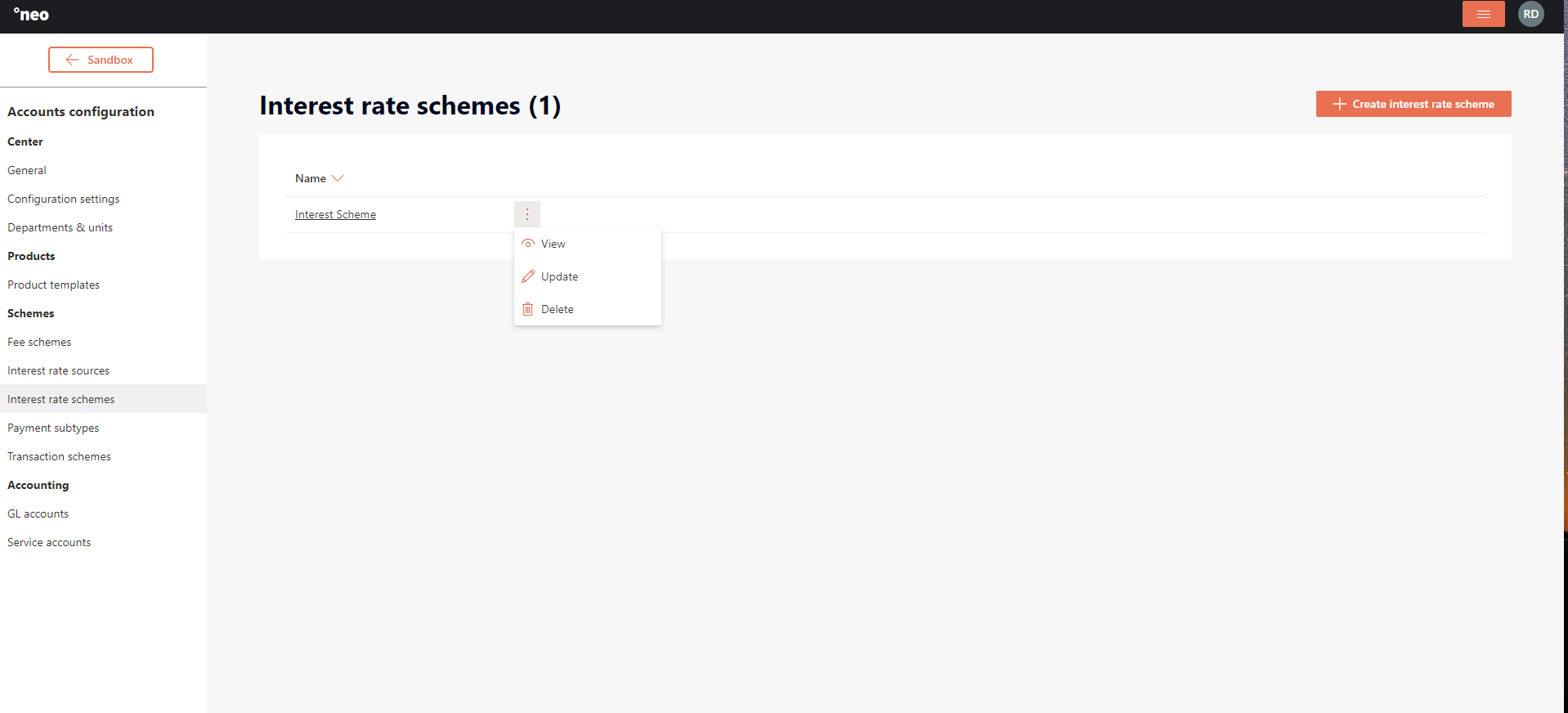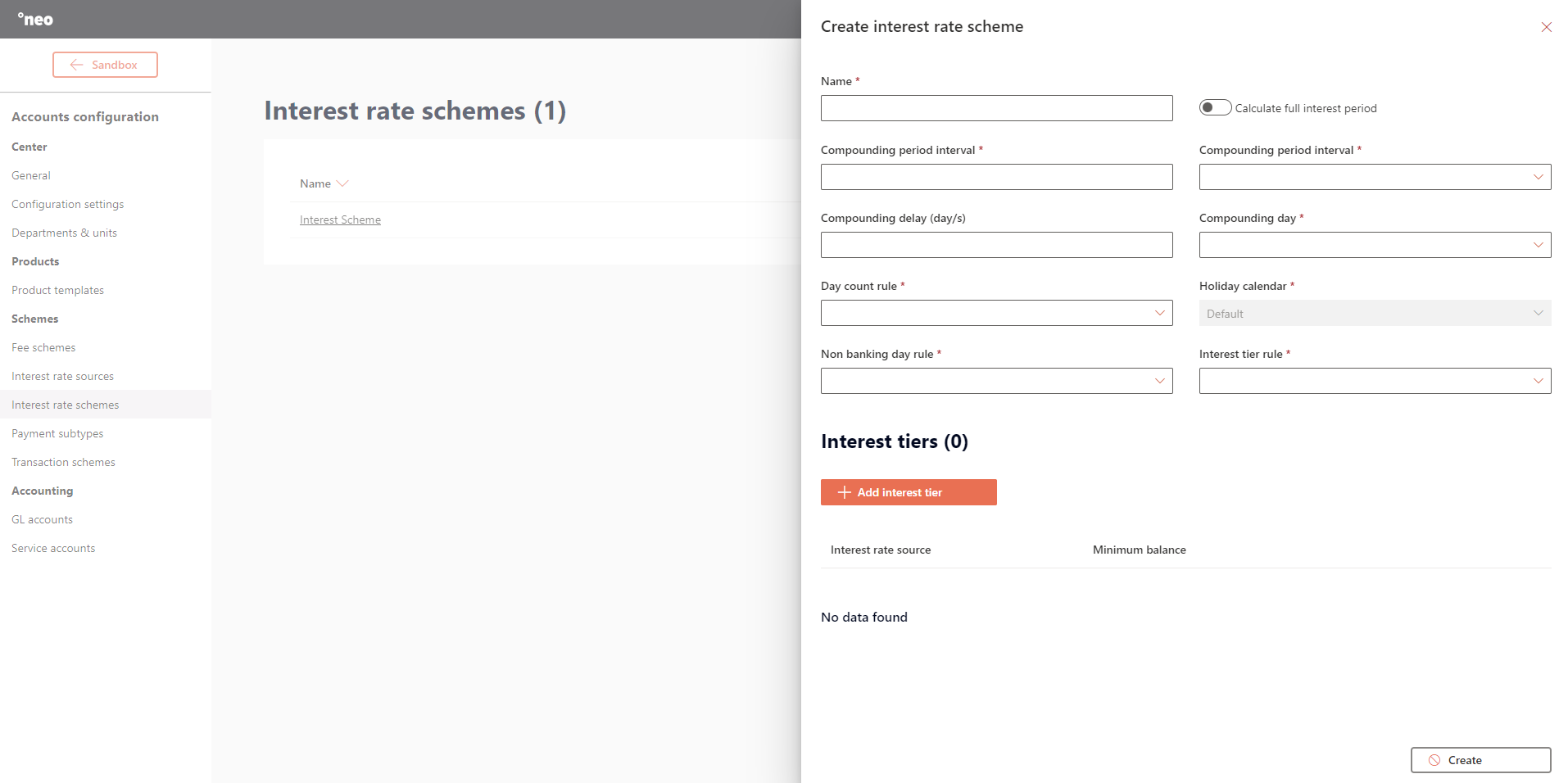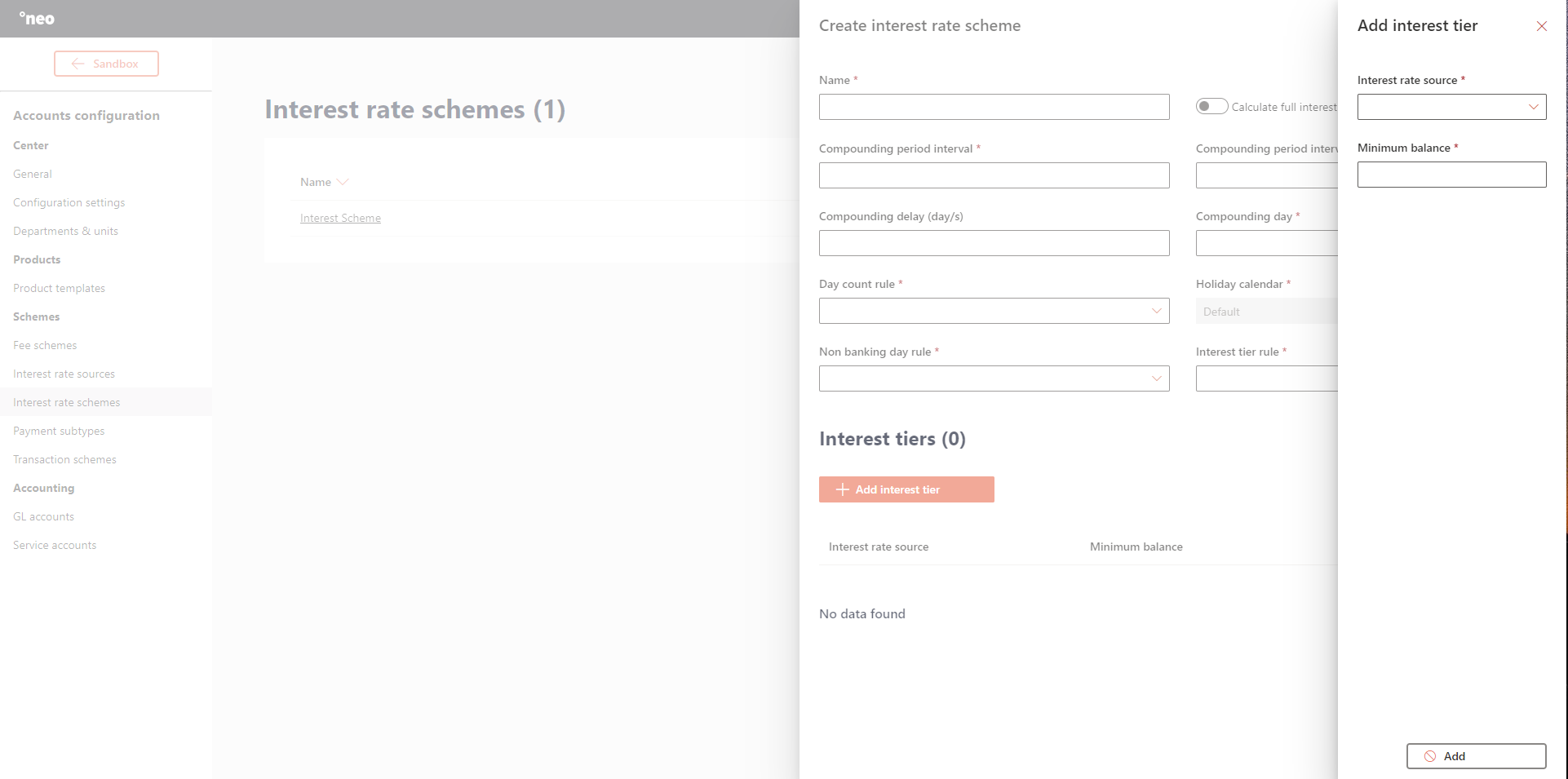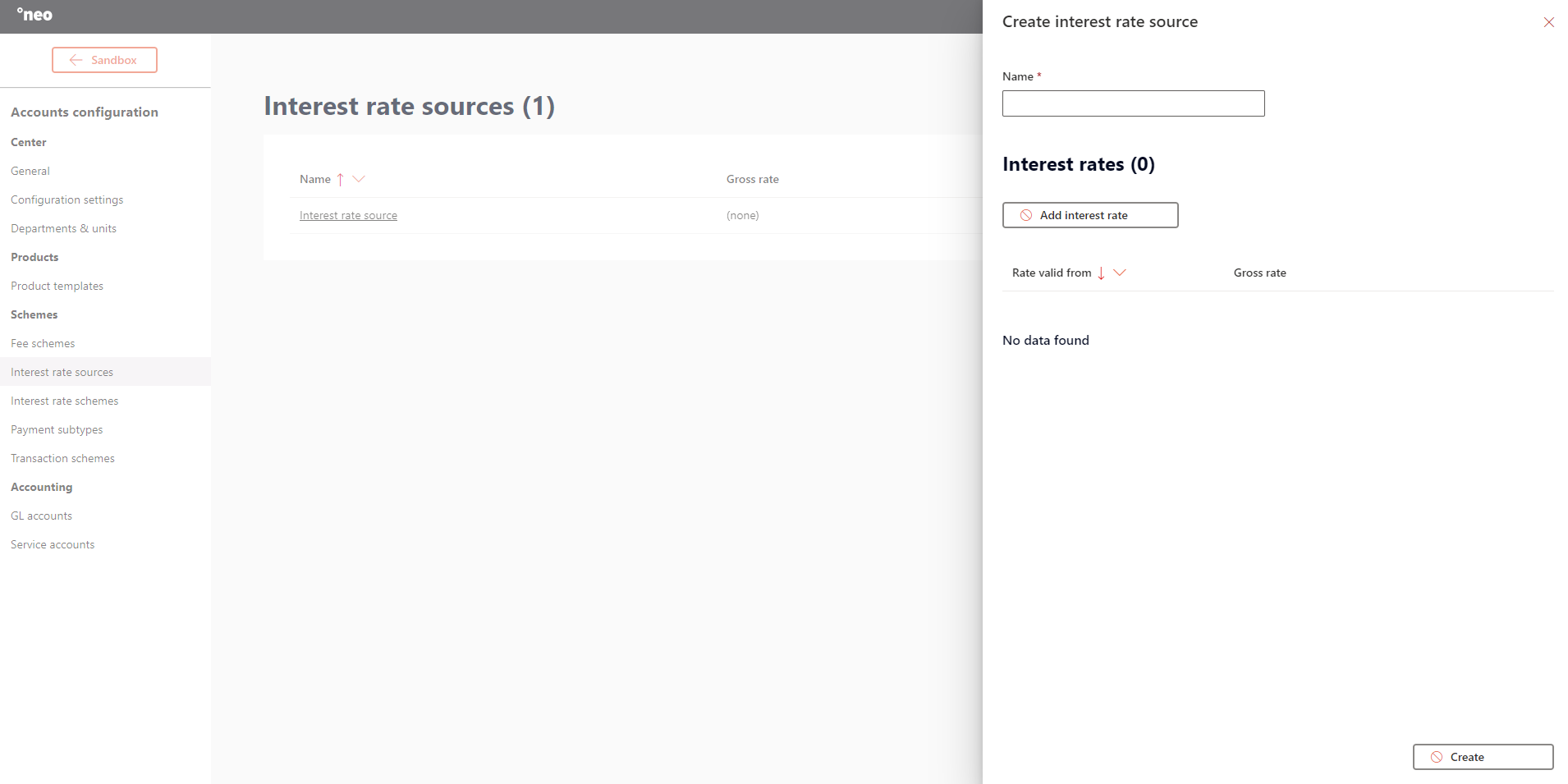# Introduction

Interest schemes are used in °neo Accounts to configure how interest for a product is calculated and paid. Therefore, an interest rate scheme is always part of a product template. New interest rate schemes can be created, updated an deleted, depending on the fact if they are used or not.An interest rate scheme relies on interest rate sources to denote the actual rate(s) that is/are applicable for the scheme.

# Configure an interest rate scheme

There are several parameters that can and must be configured for each scheme:Name

Description

Name

Denotes the name of the scheme which is referenced throughout °neo Accounts.

Calculate full interest period

Denotes if the full period is calculated in case of a delay.

Compounding period interval

The period of time between the compounding of interest (Year, month, week, days), e.g. ‘Month’ ‘6’ for a 6 month compounding period.

Compounding delay

Denotes a delay to the compounding day.

Compounding day

Denotes the compounding day.

Day count rule

Denotes the calculating rule for determining how interest accrues over time.

Holiday calendar

Always set to default, pending future functionality.

Non banking day rule

Denotes the non-banking day rule which indicates when interest payments are made to the accounts.

Interest tier rule

This rule will determine how the interest will be calculated:
• Whole Balance: the configured interest rate will applied on the whole balance
• Split Balance: the configured interest rates will applied to parts of the balance

### Interest tiers explained

Interest tiers are used to denote the percentage that is used to calculate the interest over a certain balance. These percentages are managed as part of interest rate sources. One or more tiers can be defined.Let us consider a scenario for a split balance with the following set-up:

• Interest tier 1 = 5%, Min balance = 0
• Interest tier 2 = 2%, Min balance = 30,000
• Interest tier 3 = 0%, Min balance = 1,000,000

If an accounts holds a balance of 35,000, it will get 5% interest over the first 30,000 and 2% interest on the following 5,000.
If an accounts holds a balance of 1,200,000, it will get 5% interest over the first 30,000 and 2% interest on the following 970,000 and 0% on the following 200,000.

Let us then consider a scenario for a whole balance with the following set-up:

• Interest tier 1 = 5%, Min balance = 0
• Interest tier 2 = 2%, Min balance = 30,000
• Interest tier 3 = 0%, Min balance = 1,000,000

If an accounts holds a balance of 35,000 it will get 2% interest over the full 35,000.
If an accounts holds a balance of 1,200,000 it will get 0% interest over the full 1,200,000 .

### Interest compounding explained

Compounding days
There are several options to denote the compounding day:

• First
• Last
• Recurring

Let us consider a scenario for a first day with the following set-up:

• Account opened on the 16/2
• Compounding period 3 months
• First day of the new period

The result will then be:

• The first period where the interest is paid (compounded) on: 16/2 - 31/3, and the interest will be paid on the 1/4 as part of the EOD.
• The second period where the interest is paid (compounded) on: 1/4 - 30/6, and the interest will be paid on the 1/7 as part of the EO, etc.

Let us consider a scenario for a last day with the following set-up:

• Account opened on the 16/2
• Compounding period 1 year
• Last day of the period

The result will then be:

• The period where the interest is paid (compounded) on: 16/2 - 31/12, and the interest will be paid on the 31/12 as part of the EOD.

Let us consider a scenario for a recurring with the following set-up:

• Account opened on the 16/2
• Compounding period 3 months
• Recurring

The result will then be:
The first period where the interest is paid (compounded) on: 16/2 - 16/5, and the interest will be paid on the 16/5 as part of the EOD.
The second period where the interest is paid (compounded) on: 17/5 - 16/8, and the interest will be paid on the 16/8 as part of the EOD, etc.

### Compounding delay and calculate full interest period

If the scheduled compounding date of a service is D1 and the compounding delay is n, D1 will first be adjusted according to the non-banking-day rule of the service. Then n days are added to D1. If D1+n falls on a non banking day, D1+n will be adjusted to be on the next banking day. All calculations will be the same as if the compounding was done on D1.

Scenario 1
Compounding is scheduled on the last business day of the month, and interest should be compounded for the full period, so let us consider the following set-up:

• Compounding day = Last,
• Non banking date = Previous
• Calculate full interest period = true

This would use the following calculation method:

• Let D1 be the next scheduled compounding day of a service
• Let D2 be the last day of the interest period
• Let n be the configured compounding delay
• Let D3 be D1+n (the actual system date when the compounding will be performed)
• If D1 is a non-banking-date: Let D1 be the previous banking-date before the original D1
• If D3 is a non-banking-date: Let D3 be the next banking-date after the original D3

Interest consequently is calculated and paid as follows:

• Interest is calculated until EoD D2 according to the interest-balance on D1
• Interest is compounded during EoD process when system-date equals D3
• The interest payment has the following dates:
ValueDate = D1
InterestDate = D2+1
SystemDate = D3
ClearingDate = D3
• The last compounding day of the service will be D2

Scenario 2
Compounding is scheduled on the last day of the month, independent of non-business days, so let us consider the following set-up:

• Compounding day = Last
• Non banking date = NonBusiness
• Calculate full interest period = false

This would use the following calculation method:

• Let D1 be the next scheduled compounding day of a service (Note: D1 is also the last day of the interest period)
• Let n be configured compounding delay, in days
• Let D2 be D1+n (the actual system date when the compounding will happen)
• If D2 is a non-banking-date: Adjust D2 to be the next banking date after the original D2

Interest consequently is calculated and paid as follows:

• Interest is calculated until EoD D1 according to the interest balance on D1
• Interest is compounded during EoD process when system-date equals D2
• The interest payment has the following dates:
ValueDate = D1
InterestDate = D1+1
SystemDate = D2
ClearingDate = D2
• The last compounding day of the service will be D1

# How to configure an interest rate source

Each interest rate scheme relies on interest rate sources to denote the actual percentages to calculate the interest. It is possible to register an interest rate with valid-from-date earlier than the current system date of the center. However, the valid-from-date of an interest rate being entered must be later than the latest compounding date of ANY service linked to the interest rate source.